# K vs. Coq as Language Verification Frameworks (Part 2 of 3)

Posted on December 12, 2019 by

Posted inMusab A. Alturki and Brandon Moore

In Part 1 of this post, we began by generally introducing the steps needed to verify the correctness of programs in a language verification framework, such as K and Coq. We then described how the first step, defining language syntax and program structure, can be accomplished in both K and Coq for our working example program SUM in the language IMP, highlighting the main differences between the two frameworks.

In this second part of the post, we build on the definitions introduced in the previous part and describe how the semantics of the language and testing the semantics, the second step in the process, can be achieved in K and Coq using the same example SUM. Part 3 of the series explains how the third step (specifying and verifying properties) can be done.

## Defining IMP’s Semantics

The second step in defining a formal model L of a language is to give formal semantics to its constructs. Below, we define the semantics of the language IMP.

### Execution rules in K

Semantics in K is specified by rewriting over terms, called configurations. A configuration consists of a possibly nested set of cells, where each cell represents a semantic element of the language being specified. One fundamental cell is the computation cell, which lists a sequence of computational structures defining the sequence of tasks giving semantics to a program. Other cells, such as name environments for binding constructs, threads for multi-threaded languages, heaps for dynamic memory allocation, and so on, can also be defined. The particular configuration to be defined is language-specific. IMP, for example, is a simple sequential programming language with variables, and so a simple configuration having the computation cell `<k>` and a state cell `<state>` for mapping variables to values is all what is needed: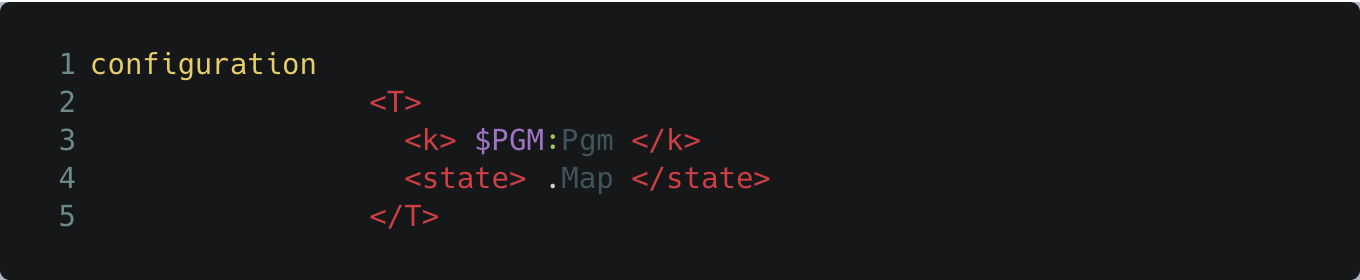The (initial) K configuration of SUM

This also defines the initial configuration of an IMP program, with the `<k>` cell having the entire program to be evaluated (in the K variable `\$PGM`) and the `<state>` cell being the empty map (denoted by `.Map`).

Transitions are specified by K rewrite rules. With the nesting structure of configurations, K rules can be specified locally where they may apply in a configuration, and only those parts of a configuration that are needed by the rules are mentioned. Configuration abstraction takes care of filling in the other parts (see the K Tutorial). For example, the semantics of replacing a variable by its value is given by the rule:The K rule defining semantics of program variables

The rule applies when the identifier `X` is to be evaluated (the computation at the top of the stack in the computation cell `<k>`). The rule matches the identifier with its corresponding mapping in the `<state>` cell and rewrites `X` to its value `I`.

For such a simple language like IMP, most of the rules do not even mention cells in the configuration, matching only on the computational structures to be reduced. For example, the semantics of `+` in IMP is given by the following rule:The K rule defining semantics of `+`

Semantics of arithmetic symbols in IMP are defined using K’s builtin operations on integers, such as `+Int` for integer addition.

The semantics of all the constructs of IMP are specified by a total of 16 rules in K (roughly one rule per construct, and only 3 rules mention cells explicitly), which can be found here.

### Execution rules in Coq

Since IMP is deterministic, i.e., every IMP program evaluates to at most one value, functions are sufficient as denotations of the language’s constructs. Relations, however, are more general and enable expressing internal steps of a computation, just as a small-step structural operational semantics definition exposes the individual steps in evaluating a program construct. More complex languages (like concurrent and multithreaded languages) are more naturally defined using relations. The rewrite arrow `=>` in K rules, for example, defines a relation.

Therefore, in Coq, the semantics of arithmetic expressions in IMP is captured by a binary transition relation on states, where a state is a pair `(AExp, Env)` consisting of an arithmetic expression `AExp` and `Env`, the current environment in which `AExp` is to be evaluated. The environment is modeled by a list of pairs associating strings (variable names) to integer constants (the only values to which variables can be mapped in IMP).

This binary transition relation can be defined in Coq as an inductively defined proposition (of type Prop),`step_e`, that tells us whether a given pair of states belongs to the relation. The following is an excerpt of the definition of `step_e` (see the full definition):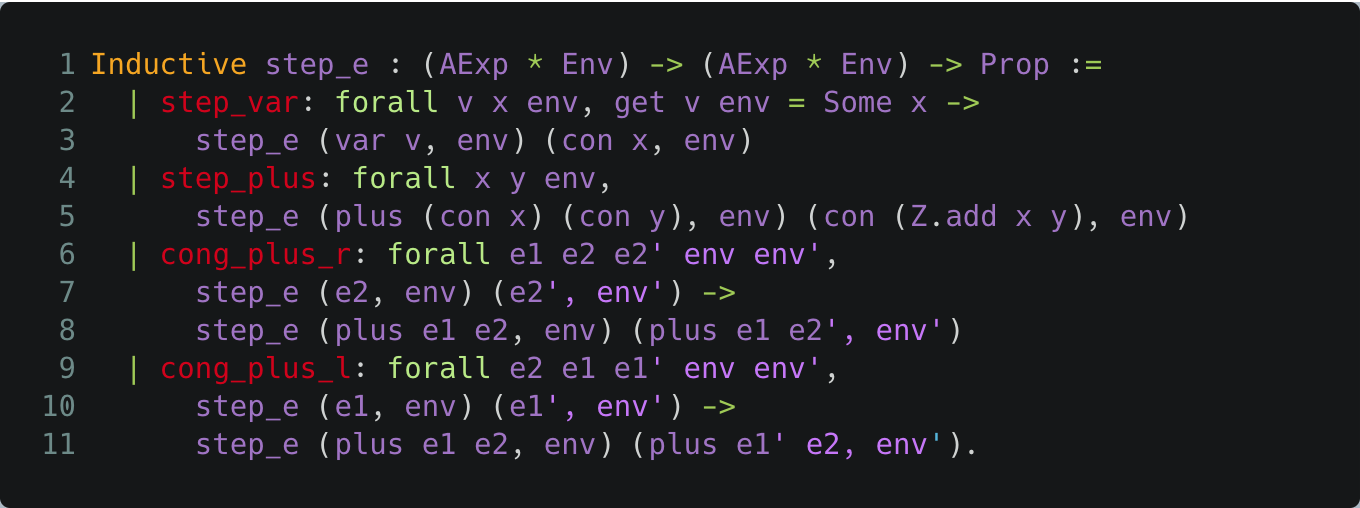The Coq rules defining semantics of arithmetic expressions

For example, `step_var` states that the program variable `var v` evaluates in one step to its value `con x` in the environment `env` (`x` is the integer value obtained from the function application `get v env`, the value to which `v` is mapped in `env`), and this holds for all program variables and environments (recall that `con` is the constructor for integer constants). Another example is the `step_plus` case, which states that any arithmetic expression of the form `plus (con x) (con y)`, in which the operands of addition have already been evaluated to constant values, evaluates in one step to the value that is the sum of the operands, regardless of the current environment (`Z.add` is the integer addition operation). Note how `step_plus`, `cong_plus_r` and `cong_plus_l` collectively specify call-by-value semantics of `plus`, which corresponds to K’s `strict` annotation highlighted above.

In addition to `step_e`, which gives semantics to arithmetic expressions, we define two more relations as inductively defined propositions `step_b` and `step_s` that give semantics to boolean expressions and statements in IMP, respectively. Their definitions follow a similar structure to `step_e`. The definition of `step_b` uses that of `step_e`, for example for defining semantics of relational operators, while the definition of `step_s` uses both `step_e` and `step_b`, for example for the conditional and iteration statements of IMP.

Finally, to give semantics to full IMP programs (variable declaration list followed by the program statement constructed with `pgm`), we introduce one more relation `step_p`, defined in terms of the relation `step_s` above. The program relation `step_p` first processes all variable declarations by augmenting the state’s environment with appropriate mappings, and then uses `step_s` to execute the program statement. Essentially, `step_p` defines the one-step semantics of IMP.

The full definition of these relations is available here.

## Testing Execution on SUM

Before getting into rigorously verifying the summation correctness property, we show how the two frameworks compare with respect to testing program executions, a necessary step in order to build confidence in the adequacy of the formal language semantics.

### Testing in K

Specifications in K are immediately executable, providing a mechanism for defining language interpreters that can be used to animate programs and evaluate language designs and language definition evolution with virtually no extra effort (beyond defining the language specification itself).

Assuming the language definition of IMP was saved as a K module in a file imp.k, calling the K compiler `kompile` on imp.k generates a parser and an interpreter for IMP that can be used to parse and execute IMP programs.

For example, for `n` initially 100, we use the SUM program given by `sum_pgm 100`, and store it in a file sum.imp. The command `kast sum.imp` outputs the K Abstract Syntax Tree (AST) of SUM. Furthermore, running the program with `krun sum.imp` outputs the final configuration resulting from executing the program, having n mapped to 0 and sum mapped to 5050 (the sum of all numbers from 1 to 100, the initial value of n).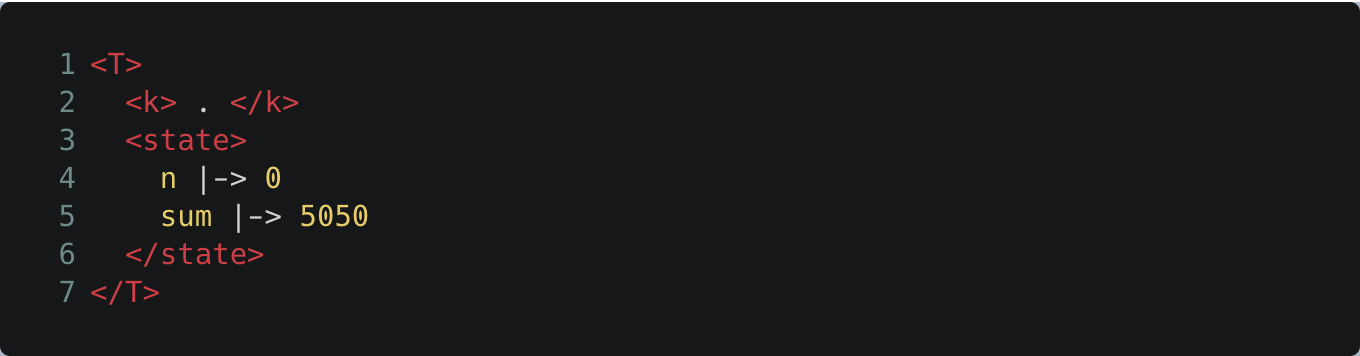The final K configuration resulting from executing SUM

Note that the `<k>` cell has the empty computation `.` indicating that the program has been fully executed.

K execution tools, especially those based on K’s LLVM backend, are optimized for execution efficiency. In fact, K’s execution with `krun` is, in some cases, faster than hand-written interpreters, such as Firefly’s K runner when compared with `ethereumjs` in `ganache-cli`.

### Testing in Coq

Coq is not designed to automatically produce an interpreter, but some machine-checked tests of how code executes under the semantics can be made by stating the example in the form of a theorem to be proved.

Recall the representation of SUM in our Coq model of IMP as a term of type `Pgm`, to which we assigned the name `sum_pgm` as follows: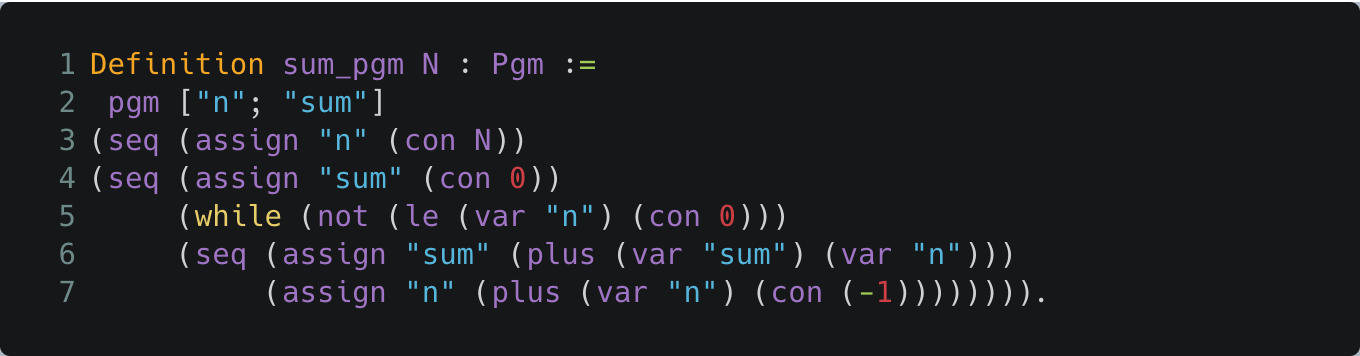Coq Definition of SUM

Using this model, we can test that SUM with `n` having the value 100, i.e. the term `sum_pgm 100`, produces the expected result by showing that `sum_pgm 100` exhibits an execution trace that begins with the state having an empty environment and ends with a state having an environment mapping `n` to 0 and `sum` to 5050: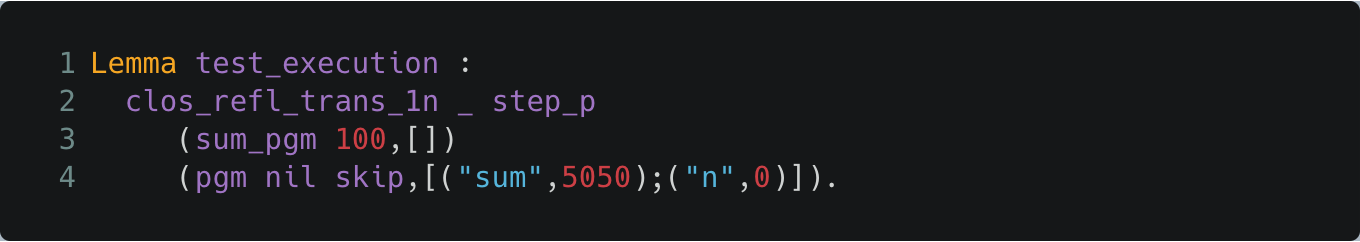Execution lemma in Coq of SUM

An execution trace is constructed by the reflexive-transitive closure of the `step_p` relation, computed by Coq’s inductively defined proposition `clos_refl_trans_1n`. Note that the program in the final state is the no-op command `skip`, requiring that the program has fully been executed.

Completing the proof requires picking the right clause of the step relation for each step of execution. Using Coq’s proof tactics to automate the search gives a certain form of automatic execution. This takes a few seconds for a hundred iterations of the loop, so performance is low, but tolerable for simple examples (the program and the proof can be found here).

Coq has other options for creating a faster interpreter with extra developer effort. Program Extraction can translate functions in the Coq language into code in other languages like OCaml or Haskell which have good native-code compilers. This still cannot automatically produce an interpreter from a transition relation defined as a predicate. The developer must write a function for taking execution steps, and ought to prove it equivalent to the relational definition.

In principle, a function could be considered the primary definition of a language, but this is likely to be less readable and harder to prove properties about. Standard practice in Coq from textbooks to large projects like CompCert is to use an inductively defined relation as the fundamental definition of a language, like we did above. Providing an alternative function for faster execution, and its correctness proof, is considered an “optimization for execution” in Coq.

#### Next-up: property verification ...

We now have a formal model of IMP defining its syntax and semantics, and a formal representation of SUM. The next and final step is to formally specify the correctness property and verify the program correct. This is described next in Part 3 of this post.